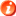P3 C31 3 TrigonalNo. 143 P3 Patterson symmetry P-3Origin on 3

Asymmetric unit 0 ≤ x ≤ 2/3; 0 ≤ y ≤ 2/3; 0 ≤ z ≤ 1; x ≤ (1 + y)/2; y ≤ min(1 - x, (1 + x)/2)
Vertices
 0, 0, 0 1/2, 0, 0 2/3, 1/3, 0 1/3, 2/3, 0 0, 1/2, 0 0, 0, 1 1/2, 0, 1 2/3, 1/3, 1 1/3, 2/3, 1 0, 1/2, 1

Symmetry operations

 (1)  1 (2)  3+   0, 0, z (3)  3-   0, 0, z

Generators selected (1); t(1, 0, 0); t(0, 1, 0); t(0, 0, 1); (2)

Positions

Multiplicity, Wyckoff letter,
Site symmetry
Coordinates Reflection conditions
General:
 3 d 1
 (1) x, y, z (2) -y, x - y, z (3) -x + y, -x, z
no conditions
Special: as above, plus
 1 c 3 . .
 2/3, 1/3, z
no extra conditions
 1 b 3 . .
 1/3, 2/3, z
no extra conditions
 1 a 3 . .
 0, 0, z
no extra conditions

Symmetry of special projections

 Along    p3a' = a   b' = b   Origin at 0, 0, z Along    p1a' = 1/2(a + 2b)   b' = c   Origin at x, 0, 0 Along    p1a' = 1/2b   b' = c   Origin at x, 1/2x, 0

Maximal non-isomorphic subgroups

 I  P1 (1) 1
 IIa none
 IIb  P32 (c' = 3c) (145);  P31 (c' = 3c) (144);  R3 (a' = a - b, b' = a + 2b, c' = 3c) (146);  R3 (a' = 2a + b, b' = -a + b, c' = 3c) (146)

Maximal isomorphic subgroups of lowest index

 IIc  P3 (c' = 2c) (143);  H3 (a' = 3a, b' = 3b) (P3, 143)

Minimal non-isomorphic supergroups

 I  P-3 (147);  P312 (149);  P321 (150);  P3m1 (156);  P31m (157);  P3c1 (158);  P31c (159);  P6 (168);  P63 (173);  P-6 (174)
 II  R3 (obverse) (146);  R3 (reverse) (146)